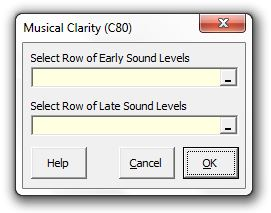### Strutt Help

Musical Clarity (C80)    1/1, 1/3

Strutt|Auditorium Acoustics|Musical Clarity (C80) calculates the objective musical clarity index C_(80) based on the early (0-80ms) and late (>80ms) components of the sound field, either measured or calculated using the Early Sound Level (Music) and Late Sound Level (Music) calculations.Strutt implements the calculation as:
C_(80)=10log((int_0^80 p^2(t)dt)/(int_80^oo p^2(t)dt)) = 10log(10^(G_(0-80)/10)/10^(G_(80-oo)/10)) = G_(0-80)-G_(80-oo)